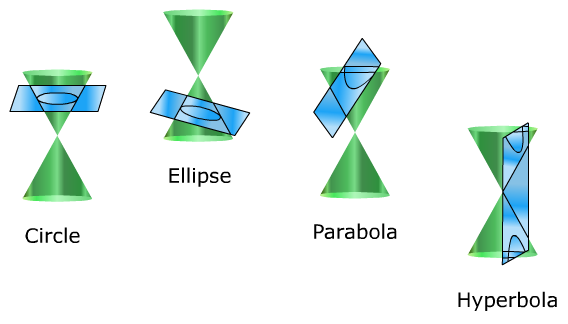# Conics: Classifying from General Equation

A conic section is the cross section of a plane and a double napped cone. The four basic conic sections do not pass through the vertex of the cone. These are:Circle - the intersection of the cone and a perpendicular plane.

Ellipse - the intersection of the cone and a plane that is neither perpendicular nor parallel and cuts through the width of the cone.

Parabola - the intersection of the cone and a plane that is neither perpendicular nor parallel and cuts through the top and a side of the cone.

Hyperbola - the intersection of a cone and a plane that is parallel to the cone and cuts through the top and bottom of the cone.

Each conic section has a unique standard equation but all of them can be described by the general equation:

GENERAL EQUATION OF A CONIC:

$A{x}^{2}+B{y}^{2}+Cx+Ey+F=0$

where A, B, C, D and E are constants.

The values of the constants A and C in the general equation reveal the type of conic the equation is describing.

CLASSIFYING A CONIC FROM GENERAL EQUATIONS:

1. Circle:          A = C

2. Parabola:     AC = 0      where A = 0 or C = 0 but not both

3. Ellipse:         AC > 0     where A and C have like signs

4. Hyperbola:   AC < 0     where A and C have unlike signs

This test only applies if the graph of the equation is a conic. It does not apply to equations that have no real graph, such as x2 + y2 = -1.

Let's use these classification rules in some examples:

Example 1: Classifying the graph of each equation.
a) $5{x}^{2}-8x+y-10=0$     b) $6{x}^{2}-2{y}^{2}+9x-3y+4=0$
 Step 1: Classify equation $5{x}^{2}-8x+y-10=0$ by determining the values of A and C and applying the general equation rule. Because the equation has no y2-term, c = 0. $5{x}^{2}-8x+y-10=0$ A = 5, C = 0 $AC=5·0=0$ Apply Rule: Because AC = 0 and both A and C are not zero, Rule 2 applies. The graph is a parabola. Step 2: Classify equation $6{x}^{2}-2{y}^{2}+9x-3y+4=0$ by determining the values of A and C and applying the general equation rule. $6{x}^{2}-2{y}^{2}+9x-3y+4=0$ A = 6, C = -2 $AC=6·-2=-12$ Apply Rule: Because AC = -12 < 0 and A and C have unlike signs, Rule 4 applies. The graph is a hyperbola.
Example 2: Classifying the graph of each equation.
a) $3{x}^{2}+3{y}^{2}-5x+2y=0$     b) $4{x}^{2}+2{y}^{2}-6y+14=0$
 Step 1: Classify equation $3{x}^{2}+3{y}^{2}-5x+2y=0$ by determining the values of A and C and applying the general equation rule. $3{x}^{2}+3{y}^{2}-5x+2y=0$ A = 3, C = 3 A = C Apply Rule: Because A = C, Rule 1 applies. The graph is a circle. Step 2: Classify equation $4{x}^{2}+2{y}^{2}-6y+14=0$ by determining the values of A and C and applying the general equation rule. $4{x}^{2}+2{y}^{2}-6y+14=0$ A = 4, C = 2 $AC=4·2=8$ Apply Rule: Because AC = 8 > 0 and A and C have like signs, Rule 2 applies. The graph is an ellipse.

 Related Links: Math algebra Component Form and Magnitude Scalar Multiplication and Vector Addition Pre Calculus

To link to this Conics: Classifying from General Equation page, copy the following code to your site: